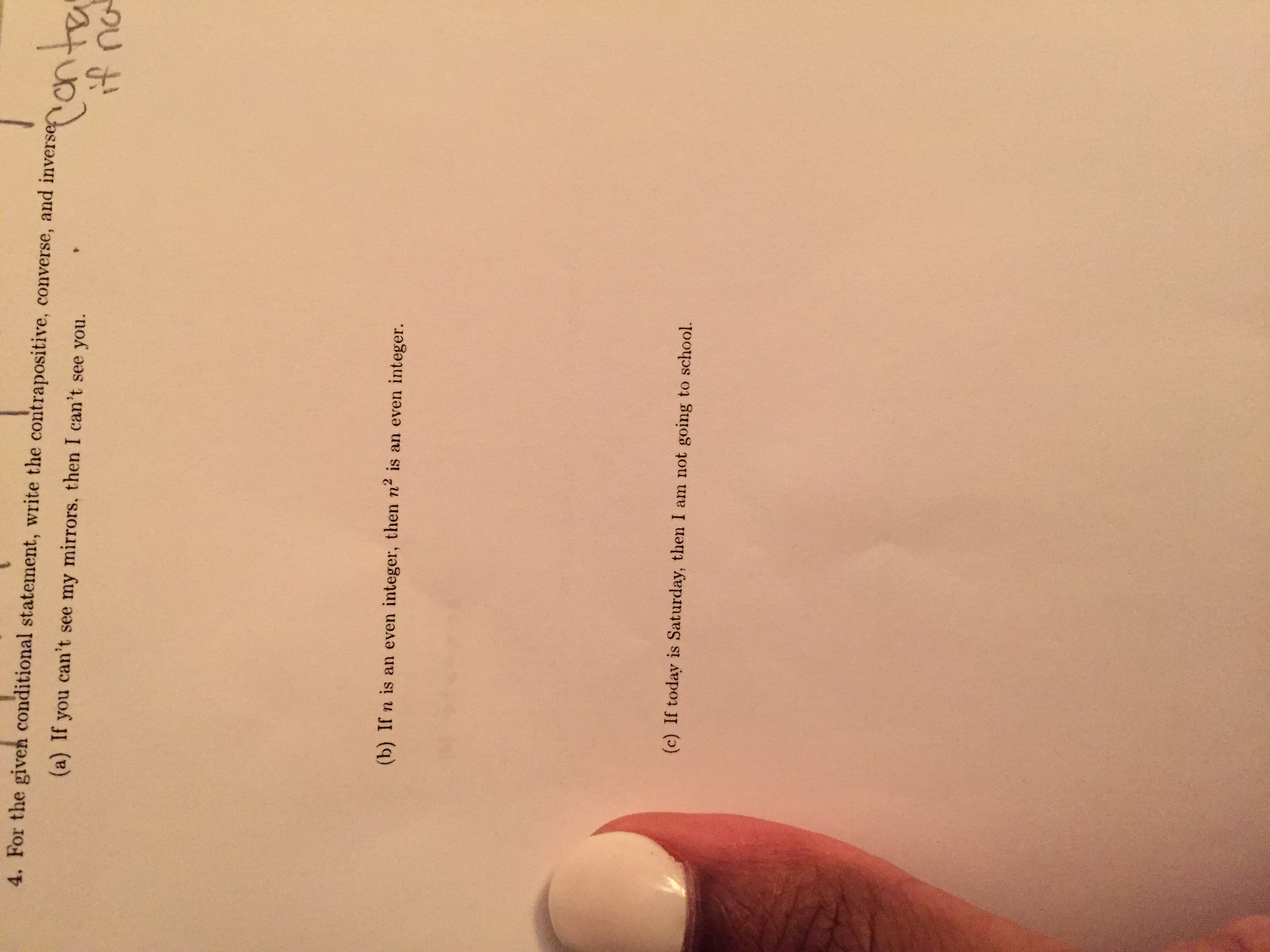# 4. For the given conditional statement, write the contrapositive, converse, and inverse n , =(a) If you can't see my mirrors, then I can't see you.(b) If n is an even integer, then n2 is an even integer.(c) If today is Saturday, then I am not going to school.

Question

For the given conditional statement write the contrapositive, converse, and inversehelp_outlineImage Transcriptionclose4. For the given conditional statement, write the contrapositive, converse, and inverse n , = (a) If you can't see my mirrors, then I can't see you. (b) If n is an even integer, then n2 is an even integer. (c) If today is Saturday, then I am not going to school. fullscreen
check_circle

Step 1

(a)

Consider the given statements:  ‘If you can’t see my mirrors, then I can’t see you’

Now, write the required statements:

Contrapositive:

It is known that contrapositive of a statement, ‘If P then Q’ is ‘If not Q then not P

Hence, the required contrapositive statement will be:

‘If I can see you, then you can see my mirrors.’

Converse:

It is known that converse of a statement, ‘If P then Q’ is ‘If Q then P

Hence, the required converse statement will be:

‘If I can’t see you, then you can’t see my mirrors.’

Inverse:

It is known that inverse of a statement, ‘If P then Q’ is ‘If not P then not Q

Hence, the required inverse statement will be:

‘If you can see my mirrors, then I can see you’

Step 2

(b)

Consider the given statements:  ‘If n is an even integer, then n2 is an even integer’

Now, write the required statements:

Contrapositive:

It is known that contrapositive of a statement, ‘If P then Q’ is ‘If not Q then not P

Hence, the required contrapositive statement will be:

‘If n2 is not an even integer, then n is not an even integer.’

Converse:

It is known that converse of a statement, ‘If P then Q’ is ‘If Q then P

Hence, the required converse statement will be:

‘If n2 is an even intege...

### Want to see the full answer?

See Solution

#### Want to see this answer and more?

Solutions are written by subject experts who are available 24/7. Questions are typically answered within 1 hour.*

See Solution
*Response times may vary by subject and question.
Tagged in

### Math